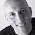## 22 June 2012

### PL/SQL Bug Confirmed from 20 June Quiz

The 20th June quiz tested your knowledge of how to remove "gaps" from a sparse array (make it dense). Daniel Kennedy posted an objection to the scoring, saying that if you use a NULL value for the index value in the collection, then none of the choices are correct.

This was a big surprise to many of us, who have long seen in our code that the idex value in an integer-indexed array cannot be NULL. Well....after checking with Bryn Llewellyn, Oracle PL/SQL Product Manager, I confirmed that this was a bug - and one that had not yet been reported!

So Bryn filed a bug report (1422744) with the explanation and code shown below.

Many aspects of the PL/SQL Challenge make me proud (the large number of players, their dedication, the growing body of knowledge about PL/SQL accessible in formats never available before, and so on). But when we identify bugs in the language or fixes required in the documentation, then I feel even more strongly how much the PL/SQL language can benefit from an active, engaged community.

Thanks, Daniel, for identifying this bug and for playing the PL/SQL Challenge.

Steven Feuerstein

v(null) := 42 succeeds when v is an IBBI – and brings Heisenberg exception handling
(from Bryn Llewellyn)

The testcase is copied below. Run it as any user with at least Create Session and Create Procedure.

The behavior of the varray and nested table tests is exactly as expected. Without the exception handling code, ORA-06502 is reported with a ORA-06512 for Line 9. And with the exception handling code, ORA-06502 is caught and nicely reported, and the program completes with no unhandled error.

However, IBBI_Test_1 runs without error and prints out 42. This is clearly a bug. Null is not a value. Rather, it's an indicator that expresses that the value is unknown.

Now it gets worse. IBBI_Test_2 simply adds the identical exception handler code for ORA-06502 around [v(null) := 42;] that Varray_Test_2 and Nested_Table_Test_2 use. But at run-time, we get this:
```
ERROR at line 1:
ORA-06502: PL/SQL: numeric or value error: NULL index table key value
ORA-06512: at "USR.IBBI_TEST_2", line 13
ORA-06512: at line 1
```

And finally, it gets worse still. IBBI_Test_3 adds more exception handling code. This time around [a := v(null);]. At run time, ORA-06502 is now obligingly caught at both [ v(null) := 42; ] and [ a := v(null); ].

```------ Testcase ------

alter session set Plsql_Warnings = 'Enable:All'
/

create procedure Varray_Test_1 authid Definer is
type t is varray(10) of pls_integer;
v t;
a pls_integer;
n pls_integer;
-- PL/SQL: numeric or value error: NULL index table key value
ORA_06502 exception; pragma Exception_Init(ORA_06502, -06502);
begin
v(null) := 42; -- Line 9
end Varray_Test_1;
/
begin Varray_Test_1(); end;
/

create procedure Varray_Test_2 authid Definer is
type t is table of pls_integer;
v t;
a pls_integer;
n pls_integer;
-- PL/SQL: numeric or value error: NULL index table key value
ORA_06502 exception; pragma Exception_Init(ORA_06502, -06502);
begin
begin
v(null) := 42;
exception when ORA_06502 then
DBMS_Output.Put_Line('ORA-06502 on "v(null) := 42"');
end;
end Varray_Test_2;
/
begin Varray_Test_2(); end;
/

create procedure Nested_Table_Test_1 authid Definer is
type t is table of pls_integer;
v t;
a pls_integer;
n pls_integer;
-- PL/SQL: numeric or value error: NULL index table key value
ORA_06502 exception; pragma Exception_Init(ORA_06502, -06502);
begin
v(null) := 42; -- Line 9
end Nested_Table_Test_1;
/
begin Nested_Table_Test_1(); end;
/

create procedure Nested_Table_Test_2 authid Definer is
type t is table of pls_integer;
v t;
a pls_integer;
n pls_integer;
-- PL/SQL: numeric or value error: NULL index table key value
ORA_06502 exception; pragma Exception_Init(ORA_06502, -06502);
begin
begin
v(null) := 42;
exception when ORA_06502 then
DBMS_Output.Put_Line('ORA-06502 on "v(null) := 42"');
end;
end Nested_Table_Test_2;
/
begin Nested_Table_Test_2(); end;
/

create procedure IBBI_Test_1 authid Definer is
type t is table of pls_integer index by pls_integer;
v t;
a pls_integer;
n pls_integer;
begin
v(null) := 42;
a := v(null);
DBMS_Output.Put_Line(a);
end IBBI_Test_1;
/
begin IBBI_Test_1(); end;
/

create procedure IBBI_Test_2 authid Definer is
type t is table of pls_integer index by pls_integer;
v t;
a pls_integer;
n pls_integer;
-- PL/SQL: numeric or value error: NULL index table key value
ORA_06502 exception; pragma Exception_Init(ORA_06502, -06502);
begin
begin
v(null) := 42;
exception when ORA_06502 then
DBMS_Output.Put_Line(q'{ORA_06502 on "v(null) := 42"}');
end; -- Line 13
a := v(null);
DBMS_Output.Put_Line(a);
end IBBI_Test_2;
/
begin IBBI_Test_2(); end;
/

create procedure IBBI_Test_3 authid Definer is
type t is table of pls_integer index by pls_integer;
v t;
a pls_integer;
n pls_integer;
-- PL/SQL: numeric or value error: NULL index table key value
ORA_06502 exception; pragma Exception_Init(ORA_06502, -06502);
begin
begin
v(null) := 42;
exception when ORA_06502 then
DBMS_Output.Put_Line(q'{ORA_06502 on "v(null) := 42"}');
end;
begin
a := v(null);
exception when ORA_06502 then
DBMS_Output.Put_Line(q'{ORA_06502 on "a := v(null)"}');
end;
DBMS_Output.Put_Line(a);
end IBBI_Test_3;
/
begin IBBI_Test_3(); end;
/
```

#### 4 comments:

1.I think you reversed "sparse" and "dense" in your opening sentence. I believe you meant "remove "gaps" from a sparse array (make it dense)".

2.Thanks, Justin. I fixed that typo.

3.Thanks for the interesting post. I'm not seeing, though, how IBBI_Test_1 runs without error and prints out 42. I get the "numeric or value error: NULL index table key value" when I run it on a couple different Oracle versions.

[Also, it looks like Varray_Test_2 is misnamed, because it uses a table of pls_integer instead of varray.]

4.Hello Anonymous,

IBBI_Test_1 does run without error only on 11gR1 and higher,
on 10g it behaves as expected.

Best Regards,
Iudith